# binscatter

Binned scatter plot

## Syntax

``binscatter(x,y)``
``binscatter(x,y,N)``
``binscatter(___,Name,Value)``
``binscatter(ax,___)``
``h = binscatter(___)``

## Description

example

````binscatter(x,y)` displays a binned scatter plot for vectors `x` and `y`. A binned scatter plot partitions the data space into rectangular bins and displays the count of data points in each bin using different colors. When zooming into the plot, the bin sizes automatically adjust to show finer resolution.```

example

````binscatter(x,y,N)` specifies the number of bins to use. `N` can be a scalar or a two-element vector `[Nx Ny]`. If `N` is a scalar, then `Nx` and `Ny` are both set to the scalar value. The maximum number of bins in each dimension is 250.```
````binscatter(___,Name,Value)` specifies property values with one or more name-value pair arguments. For example, you can specify `'ShowEmptyBins'` as `'on'` to color areas with no data points. For a full list of properties, see Binscatter Properties. Use this option with any of the input argument combinations in previous syntaxes.```
````binscatter(ax,___)` plots into the axes specified by `ax` instead of into the current axes (`gca`). The `ax` input can precede any of the input argument combinations in previous syntaxes.```

example

````h = binscatter(___)` returns a `Binscatter` object. Use this object to inspect and adjust the properties of the binned scatter plot.```

## Examples

collapse all

Generate random numbers in both the x and y dimensions and create a binned scatter plot. The `binscatter` function automatically chooses an appropriate number of bins to cover the range of values in the data.

```x = randn(1e6,1); y = 2*x + randn(1e6,1); binscatter(x,y)```Plot a binned scatter plot of 10,000 random numbers sorted into 30 bins in the x dimension and 10 bins in the y dimension.

```rng default % for reproducibility x = randn(1e4,1); y = randn(1e4,1); h = binscatter(x,y,[30 10]);```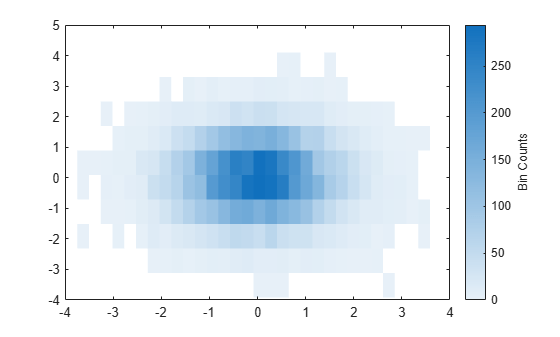Find the bin counts. The result is a matrix with the top left element corresponding to the bin count of the bottom left bin in the plot. The x bins are in the rows of the matrix and the y bins are in the columns.

`counts = h.Values;`

Create a binned scatter plot of some random data points.

```x = randn(1e5,1); y = randn(1e5,1); binscatter(x,y)```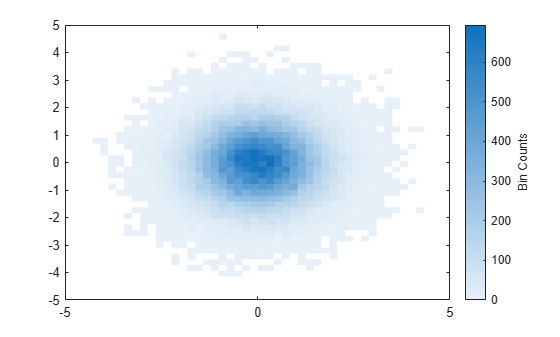The default color map ranges from light colors (for small values) to dark colors (for large values). Switching to a color map that uses dark colors for small values can make it easier to spot outliers.

Use the `colormap` function to change the colors in the plot. Pass in the current axes handle using `gca`.

`colormap(gca,'parula')`Generate 1,000 random numbers and create a binned scatter plot. Return the `Binscatter` object to adjust properties of the plot without recreating the entire plot.

```x = randn(1000,1); y = randn(1000,1); h = binscatter(x,y)```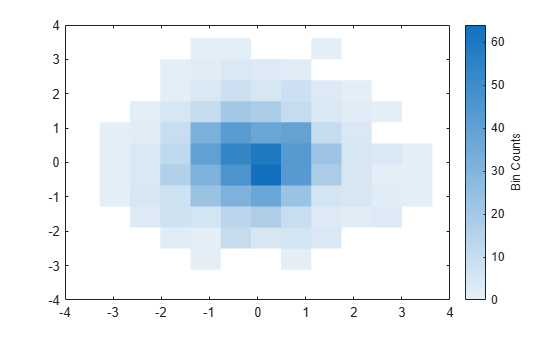```h = Binscatter with properties: NumBins: [11 11] XBinEdges: [-3.2764 -2.6485 -2.0206 -1.3927 -0.7648 -0.1369 0.4910 ... ] YBinEdges: [-3.1155 -2.5034 -1.8914 -1.2794 -0.6674 -0.0553 0.5567 ... ] Values: [11x11 double] XLimits: [-3.2764 3.6305] YLimits: [-3.1155 3.6168] FaceAlpha: 1 Show all properties ```

Specify exactly how many bins to use in each direction.

`h.NumBins = [20 30];`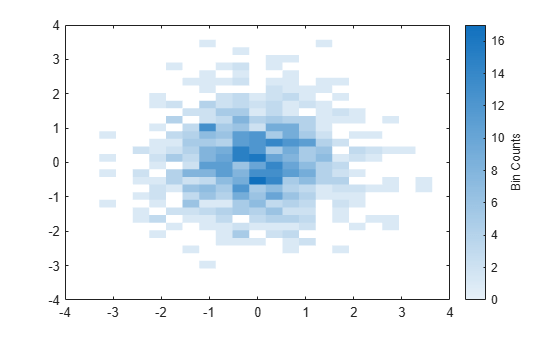Turn on the display of empty bins in the plot.

`h.ShowEmptyBins = 'on';`Specify the extent of the axes with the `XLimits` and `YLimits` properties. Then limit the bin limits in the x direction with a vector.

```xlim(gca,h.XLimits); ylim(gca,h.YLimits); h.XLimits = [-1 1];```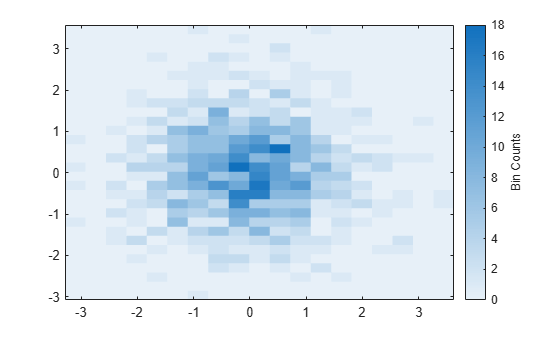## Input Arguments

collapse all

Input vectors, specified as real vectors of the same length.

Data Types: `single` | `double` | `int8` | `int16` | `int32` | `int64` | `uint8` | `uint16` | `uint32` | `uint64` | `logical` | `datetime` | `duration`

Number of bins, specified as a scalar or two-element vector ```[Nx Ny]```.

• If `N` is a two-element vector ```[Nx Ny]```, then `binscatter` uses `Nx` bins in the x dimension and `Ny` bins in the y dimension.

• If `N` is a scalar, then `Nx` and `Ny` are both set to the scalar value.

`binscatter` uses `Nx` and `Ny` bins along the x and y dimensions in the initial plot, when the axes are not zoomed in. (The axes are not zoomed in when the `XLimMode` and `YLimMode` properties are both `'auto'`.) When zooming, `binscatter` adjusts the number of bins to maintain a bin size such that the visible portion of the plot is approximately divided into `Nx`-by-`Ny` bins.

The maximum number of bins in each dimension is 250. The default number of bins is computed based on the data size and standard deviation and does not exceed 100.

Example: `[10 20]`

Data Types: `single` | `double` | `int8` | `int16` | `int32` | `int64` | `uint8` | `uint16` | `uint32` | `uint64`

Target axes, specified as an `Axes` object. If you do not specify the axes, then `binscatter` uses the current axes (`gca`).

### Name-Value Arguments

Specify optional pairs of arguments as `Name1=Value1,...,NameN=ValueN`, where `Name` is the argument name and `Value` is the corresponding value. Name-value arguments must appear after other arguments, but the order of the pairs does not matter.

Before R2021a, use commas to separate each name and value, and enclose `Name` in quotes.

Example: `binscatter(x,y,'ShowEmptyBins','on')` turns on the display of empty bins in the plot.

The properties listed here are only a subset. For a complete list, see Binscatter Properties.

Data limits in x-dimension, specified as a two-element vector `[Xmin Xmax]`.

`binscatter` only displays data points that fall within the specified data limits inclusively, ${X}_{\mathrm{min}}\le X\le {X}_{\mathrm{max}}$.

Example: `[0 10]`

Data Types: `single` | `double` | `int8` | `int16` | `int32` | `int64` | `uint8` | `uint16` | `uint32` | `uint64` | `datetime` | `duration`

Data limits in y-dimension, specified as a two-element vector `[Ymin Ymax]`.

`binscatter` only displays data points that fall within the specified data limits inclusively, ${Y}_{\mathrm{min}}\le Y\le {Y}_{\mathrm{max}}$.

Example: `[0 10]`

Data Types: `single` | `double` | `int8` | `int16` | `int32` | `int64` | `uint8` | `uint16` | `uint32` | `uint64` | `datetime` | `duration`

Toggle to show empty bins, specified as either `'off'` or `'on'`, or as numeric or logical `1` (`true`) or `0` (`false`). A value of `'on'` is equivalent to `true`, and `'off'` is equivalent to `false`. Thus, you can use the value of this property as a logical value. The value is stored as an on/off logical value of type `matlab.lang.OnOffSwitchState`.

Specify `'on'` or `true` to color tiles in the plot that fall within the bin limits, but have no data points.

## Output Arguments

collapse all

`Binscatter` object. Use this object to inspect and adjust properties of the plot. For a full listing of properties, see Binscatter Properties.

## Tips

• Change the `ColorScale` property of the axes to `'log'` to produce better bin coloring when a few bins dominate the plot.

```ax = gca; ax.ColorScale = 'log';```

## Version History

Introduced in R2017b